# Monte Carlo simulation

Monte Carlo uses statistical random sampling to calculate values for an analyzed dimension, including mean, sigma, accuracy, largest/smallest value, and percent in tolerance.

General use of the Monte Carlo Simulation tool is described below.

## Setting up a Monte Carlo simulation

The methods for adding derived parameters (dimensions and variables) and their contributors (non-derived dimensions and constraints) to a Monte Carlo simulation are described below.

When initiating the Monte Carlo simulation setup, you can:

• Select stack(s) and let Concept automatically detect contributors to those stack(s) and add all contributors to the simulation setup

• Select stack(s) and contributors prior to the simulation setup, limiting the contributor list to only the selected contributors

After initiating the setup using one of the above methods, you can add and remove individual stacks and contributors at any time.

## Adding individual objects to an existing Monte Carlo Simulation setup

To add additional items to a Monte Carlo simulation setup:

1. Open the Monte Carlo tool in the Modules view.

2. Select the objects from the sketch or parameter table, and click Add Selected Objects under the Setup area of the Monte Carlo Simulation dialog. Your selected derived dimensions are added to the Stacks area, and non-derived dimensions and constraints are added to the Contributors table.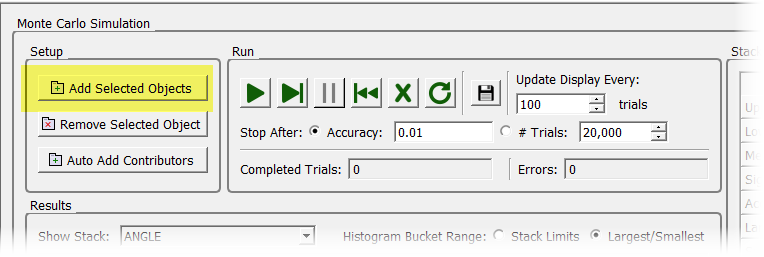3. You may continue to select individual contributors and add them by clicking Add Selected Objects. (Note: Enventive Concept does not check whether the contributors you add individually are in fact contributing to the variation of the stacks.)

### Determining which contributors to add manually

To add contributors individually when working in an assembly, you may need to select All Models under the View area in the Math view (set to Active Model by default). This will show you all the parameters for all models in the assembly.

You may want to run tolerance analysis on the dimensions you are analyzing to determine the contributors to add to Monte Carlo analysis.

### Adding all contributors to an existing setup

In an existing Monte Carlo simulation setup, you can automatically detect all contributors to the stack(s) and add them to the Contributors table at any time. To do this, click Auto Add Contributors.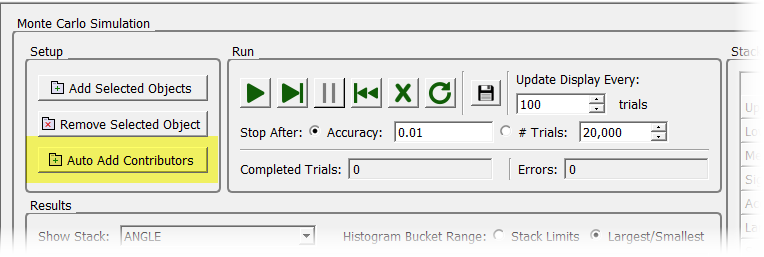### Removing individual objects from an existing setup

In an existing Monte Carlo simulation setup, you can automatically remove individual stacks or contributors at any time. To do this, select the object from the Stacks or Contributors table, and then click Remove Selected Object.

Note: Only single objects can be selected for removal; you cannot select multiple objects using the Ctrl or Shift key. If you need to remove many objects, you may want to consider clearing the setup and creating a new setup. For example, you may want to determine which contributors to include in the setup and manually add those contributors, as described above.

## Running a Monte Carlo analysis

After setting up the Monte Carlo simulation (as described in the previous section), you can run the analysis as many times as you like. The time required to run the analysis depends primarily on the size of the model and may increase or decrease depending on your settings, as noted below.

You can change the following settings in the Run area before running the Monte Carlo analysis:

• Switch between stopping the simulation after the specified accuracy of mean is reached or after a specified number of trials is reached.
• Accuracy: Select this option to run the simulation until the specified accuracy is reached. Decreasing this value increases the time to run the simulation and improves its accuracy, while increasing the value has the opposite effect.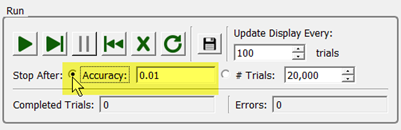Based on the samples that have already been run, Enventive Concept computes the mean value of the results and a 95% confidence interval for the mean, based on a Student’s t-test. The accuracy value that you specify is the desired range for the 95% confidence interval, where the units for “accuracy” are the same as the units of the analyzed parameter.

For example, say you have a simulation set up to reach an accuracy level of 0.010 for an analyzed parameter, and when you run the simulation it stops at a mean value of 10.316. This means that you can assume (with 95% confidence) that the mean value will be between 10.311 and 10.321.

• Trials: Select this option to run the simulation until the specified number of trials is reached. Increasing this value increases the time to run the simulation and improves its accuracy, while increasing the value has the opposite effect.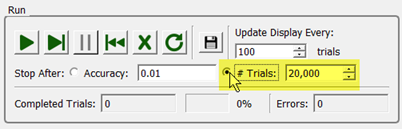You can change any of the following settings in the Run or Results areas before running or during running the Monte Carlo analysis:

• Update display (per specified number of trials): Decreasing this value generally increases the time to run the simulation, but may allow you to better see the effects of the simulation as it is running. This setting does not affect the accuracy of the simulation.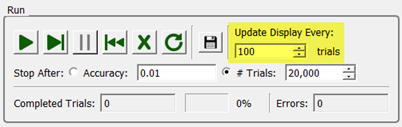• Show Stack: Lets you select which stack to view histogram results for.

• Histogram bucket range: When Largest/Smallest is selected, the histogram displays all results and how the limits fit into the range of results. When Stack Limits is selected, the x axis is bound by the stack limits.

### To run the analysis:

1. Press Run to run the entire analysis: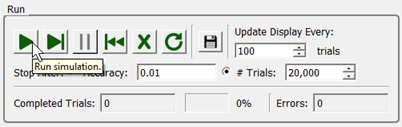or

press Single Step to run the analysis one step at a time:2. Press Pause at any time while running the entire analysis (does not apply to running the analysis using single steps). Note that the cursor appears as a working (hourglass) icon while running the analysis, but you can still select the Pause button.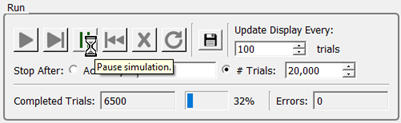For example, after you begin running the analysis, you can investigate a particular stage of the analysis by pressing Pause and then clicking Single Step to see details. Note that the simulation will not pause in a perturbed state.

3. If desired, save the current results of the analysis.
4. After running all or part of the analysis, you have the following options:
• Refresh: Rescans the model to get current Stack nominals and contributor distribution values.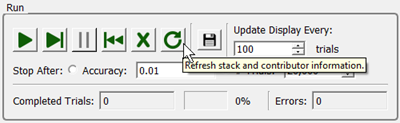• Clear results only: Clears the analysis results, but does not remove the stacks and contributors from the simulation setup. For example, you may want to adjust the simulation's settings, such as changing the accuracy level, and then rerun the analysis on the same stacks again.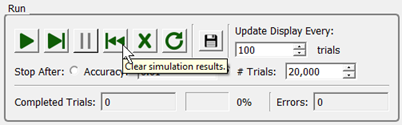• Clear all stacks and contributors: Clears all stacks and contributors from the Monte Carlo simulation. Use this option to run a new analysis.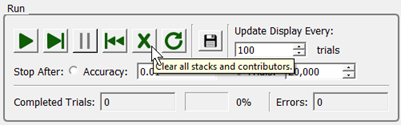For example, if contributor values change and you want to rerun the simulation, first use Refresh, then use Clear results, then rerun the analysis.

## Analysis results

The analysis results look similar to the following illustration when complete.

### Goodness of fit (sample moments and Pearson distribution data)

Monte Carlo Simulation results include "goodness of fit," which tests how well the analyzed data fits the selected distribution type.

Sample moments and Pearson distribution analysis is performed to determine whether output data may be considered Normal/Gaussian or whether a different Pearson distribution type provides a better fit. Depending on the determined distribution type, appropriate distribution parameters are displayed; i.e., Mean, Sigma, and Cpk or Mean, Variance, Skew, and Kurtosis.

The "goodness of fit" data is shown at the bottom of the Monte Carlo report after running an analysis:

## Analyzing contributor groups

For contributor groups (repetitive size dimensions, constant thickness dimensions, and line to line constraints), all the dimensions in the set share the same distribution because they all share the same tolerance specification. All parts of the contributor are perturbed independently (as is done in standard tolerance analysis), regardless whether the parts are in the contributor list. This may affect the stack data.

For example, if a repetitive size dimension is a contributor, then the Monte Carlo analysis will perturb all the dimensions in the set, according to their distribution. For hole pattern constraints, Enventive Concept only perturbs the location of the hole patterns, not their orientation.

## Analyzing multiple instances from the same master model

When you run Monte Carlo analysis on an assembly that contains multiple instances of a master model, the instances are perturbed in unison. If the instances need to vary independently, you must branch the instances so they are associated with different master models.

## Saving Monte Carlo results to a file

You can save the resulting nominals from each trial (contribution values from each trial are not saved) to a comma-separated file, in .csv format by default. Files may also be saved in other formats such as .txt or .dat. You can save the results at any time; for example, if you pause the analysis, you can save the results that are completed at the time you pause.

### To save the analysis:

1. Click Save in the Run area.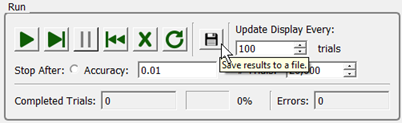2. In the Save Monte Carlo Results dialog that appears, specify a name and location for the file.
3. Change the extension if needed and save the file.

## Specifying distribution type and parameters

Monte Carlo analysis uses Gaussian distribution by default. You can optionally use various other distribution types. Note that modifying Monte Carlo distribution types is an advanced capability that relies on manufacturing process distribution information.

### To designate a distribution type for a contributor:

1. Select the contributor.
2. In the Properties Explorer's Tolerance area, select User-specified under the MC Distrib field.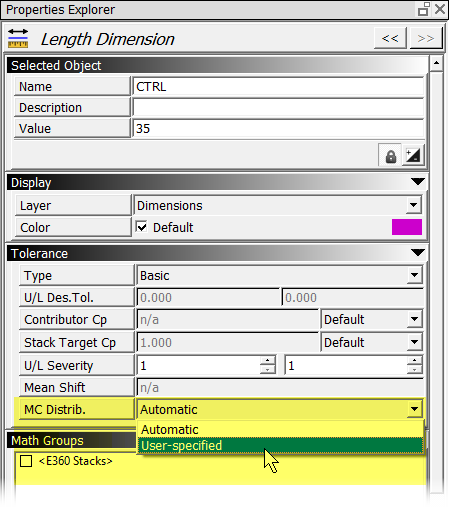3. Under Monte Carlo Distribution Parameters, select the desired distribution type and change its settings (described in the next section) if needed. (Note that only the distribution types that are relevant to the selected contributor are listed for selection. The following illustration does not show all distribution types.)### Distribution types

The following distribution types are available for use with Monte Carlo simulation. Enventive Concept applies default specifications for each distribution type, all of which you can modify as needed (except as noted below).

 Distribution Type Specifications Gaussian Mean Standard deviation Uniform Max Min Triangular Max Peak Min Weibull Location Shape Scale 2D Gaussian (For concentric circles and position constraints only.) Mean (X) Mean (Y) Bias Angle Standard deviation (X) Standard deviation (Y) 2D Circular Uniform (For concentric circles and position constraints only.) Mean (X) Mean (Y) Diameter 2D Circular Gaussian (For concentric circles and position constraints only.) Mean (X) Mean (Y) Standard deviation 2D Pin in Hole (For Pin-in-Hole constraints only.) N/A (Calculations are done automatically and cannot be modified.) Line-Line Gaussian (For line-to-line constraints only.) Mean Standard deviation Line-Line Uniform (For line-to-line constraints only.) Max Min Parallelism Truncated Gaussian Mean Standard deviation Max Min Trapezoidal Max Upper Mode Lower Mode Min

Notes:

• The 2D Uniform distribution type can be used for line-to-line dimensions, including cylindrical/rectangular feature of size (FOS) dimensions. (The 2D Gaussian and 2D Uniform distribution types for line-to-line dimensions represent the effects of both Size and Orientation variation.)
• For concentric circles and position constraints, you can use 2D Gaussian, 2D Circular Uniform, or 2D Circular Gaussian, for which you can enter the mean x and mean y values (e.g., from a CMM machine or similar).